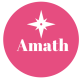# What are good math apps?

By Yuefei Su, CEO of Amath

Mathematics apps are used more and more widely in learning. Commonly used math apps are as follows:

1. Bedtime Math, the website will publish a math question in the form of a story for players to answer every day. For young children, the problem obtained is simple addition and subtraction, which can usually be solved in one step; for teenager, multiplication and division need to be completed, and more steps are required.
2. Dragon Box Algebra, the operation process is gradually increasing the difficulty. At the beginning, you only need to click and drag to operate, and use some card games to explain the concept of algebra to children. Subconsciously, it will increase the difficulty of the game. Let children solve some algebraic problems by themselves, such as solving X. Here, complex algebraic operations are transformed into interesting game rules, which are easier for children to accept and learn.
3. Motion Math, this APP can help children learn a variety of math skills, including addition, subtraction, multiplication, division, word problems, fractions, number lines, decimals, negative numbers, and so on.
4. Twelve a Dozen-all access, the whole game is run through by a character named “Twelve”. The plot of the game is set to destroy Dozenopolis. Players need to help Twelve cross the town and pass 30 levels to rescue her family. . In this process, they need to overcome many math-related challenges, including addition, subtraction, multiplication, and division.
5. Mystery Math Town, in the game, the children’s task is to help find fireflies, which are trapped in jars and hidden in houses in the mysterious math town. In order to find fireflies, they need to move between different houses in the town to collect more fireflies. When entering the house, there will be a number of math-related questions and levels. Only when these math problems are solved can you enter the room to collect fireflies.
6. Slice Fractions, the learning of this game is mainly divided into three stages, each stage has corresponding questions, the difficulty gradually increases, and different mathematical knowledge is involved. In the first stage, children learn how to divide a shape into equal parts, and then compare it with an overall shape to get a preliminary exposure to the concept of fractions. In the second stage, start to learn the numerator and denominator in detail. The third stage helps children learn how to sort scores and learn simple addition and subtraction of scores.
7. Math Snacks, a combination of interesting videos and games are presented to children, through animation to illustrate the concept of mathematics, and games to help children understand mathematical knowledge, complement each other, and master a certain knowledge point.
8. Get The Math, a website that proves to children how to use mathematics, shows how professionals from all walks of life apply mathematics to music, fashion, video games, restaurants and other fields through short videos.
9. Amath, a gamification practice APP, uses gamification incentive mechanism to stimulate children’s learning motivation, allowing children to practice in the game, so that the practice is no longer painful and uncomfortable. The practice content is synchronized with the knowledge points of the course examination, which is suitable for children’s self-adaptive self-study and study guidance, so as to familiarize themselves with the knowledge points and improve the test scores.## Author: amath

Learn math from amath.